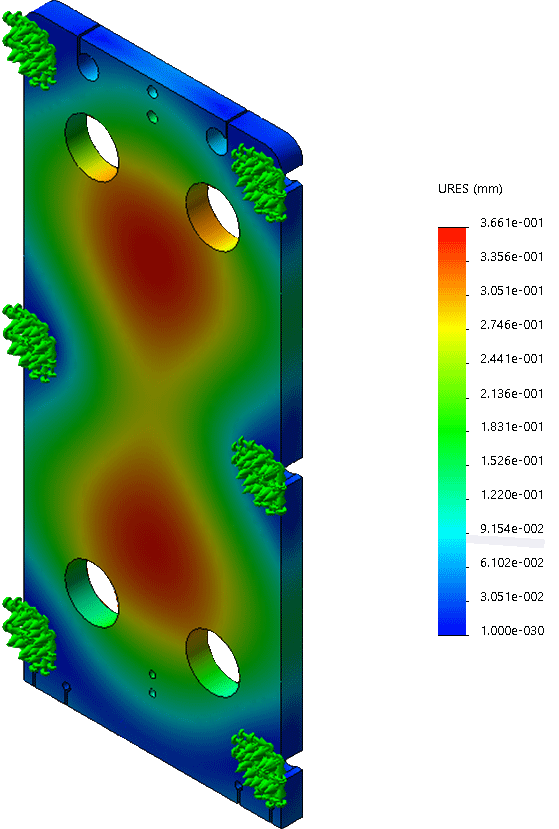## - Working Principle

Channels are formed between the plates and the corner ports are arranged so that two media flow through alternate channels. The heat is transferred through the plate between the channels, and complete counter-current flow is created for highest possible efficiency. The corrugation of the plates provides the passage between the plates, supports each plate against the adjacent one and enhances the turbulence, resulting in efficient heat transfer.

## - Software Design

The plate heat exchangers design according to technical specifications is calculated with HFM PHE software by which can manipulate the medium’s physical properties and thermal length of the plate heat exchanger. We design the economic and reliable solution of HFMPHE™ plate pattern combination to cater for various operating specifications with high efficiency.

## - Required Parameters

The main parameters for a plate heat exchanger design include total thermal load, inlet and outlet temperatures of hot side, inlet and outlet temperatures of cold side, flow rates of hot and cold sides. The 5 out of 7 parameters are necessary to make the calculation design, and at least 2 parameters at least of each side ( hot or cold ) are necessary to make the design.

## - Pressure Loss

The other influential parameter of a plate heat exchanger design is pressure loss. The number of pressure loss results in the selection of plate pattern/ model and plate quantity/ how many pieces. In addition, the thickness of frame plates are impacted by the actual operation pressure of the equipment.

## - Plate Heat Exchanger Design Procedures1.Calculate duty, the rate of heat transfer required.

2.If the specification is incomplete, determine the unknown fluid temperature or fluid flow rate from a heat balance.

3.Calculate the log mean temperature difference, ΔTlm.

4.Determine the log mean temperature correction factor, Ft.

5.Calculate the corrected mean temperature difference ΔTm = Ft x ΔTlm.

6.Estimate the overall heat transfer coefficient.

7.Calculate the surface area required.

8.Determined the number of plates required = total surface area/area of one plate.

9.Decide the flow arrangement and number of passes.

10.Calculate the film heat transfer coefficients for each stream.

11.Calculate the overall coefficient, allowing for fouling factors.

12.Compared the calculated with the assumed overall coefficient. If satisfactory, say -10% to +10% error, proceed. If unsatisfactory, return to step 8 and increase or decrease the number of plates.

13.Check the pressure drop for each stream.